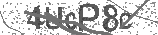﻿ Tension Force Physics Problems | Safe Videos for Kids
Welcome

# Tension Force Physics Problems

Thanks! Share it with your friends!

URL

You disliked this video. Thanks for the feedback!

Sorry, only registred users can create playlists.URLChannel: The Organic Chemistry Tutor
Categories: Physics   |   ScienceFind Related Videos  added
25 Views## Description

This physics video tutorial explains how to solve tension force problems. It explains how to calculate the tension force in a rope for a object descending with a downward acceleration using newton's laws of motion. it also discusses how to use free body diagrams and the weight force to calculate the tension force in two ropes at different angles. There's another tension force physics problem that asks you to calculate the tension in two cables / ropes where one rope is in the horizontal direction and the second rope is at an angle.

Full 1 Hour Video:
https://www.patreon.com/MathScienceTutorBe the first to comment